# Telangana SSC Board (TS SSC) Question Paper 2 for Class 10th Maths 2016 In PDF

## Telangana 10th Annual Exam Question Papers 2016 Maths Paper 2 with Solutions – Free Download

Telangana SSC Class 10 Maths 2016 Question Paper 2 with solutions can be accessed at BYJU’S, which are prepared by the expert faculty. Different patterns of questions appear in Telangana SSC exams, which test the students’ ability to understand the concepts of Maths. For more knowledge on Class 10 Maths concepts, students can visit BYJU’S. Also, they can get all TS SSC Class 10 Maths previous year papers along with the solutions. Students can also get other years’ question papers of TS SSC Class 10 Maths, so that they can practice them before going to attempt the main exam. This will help the students in overcoming stress and boost confidence over the exam.

### Telangana Board SSC Class 10 Maths 2016 Question Paper 2 with Solutions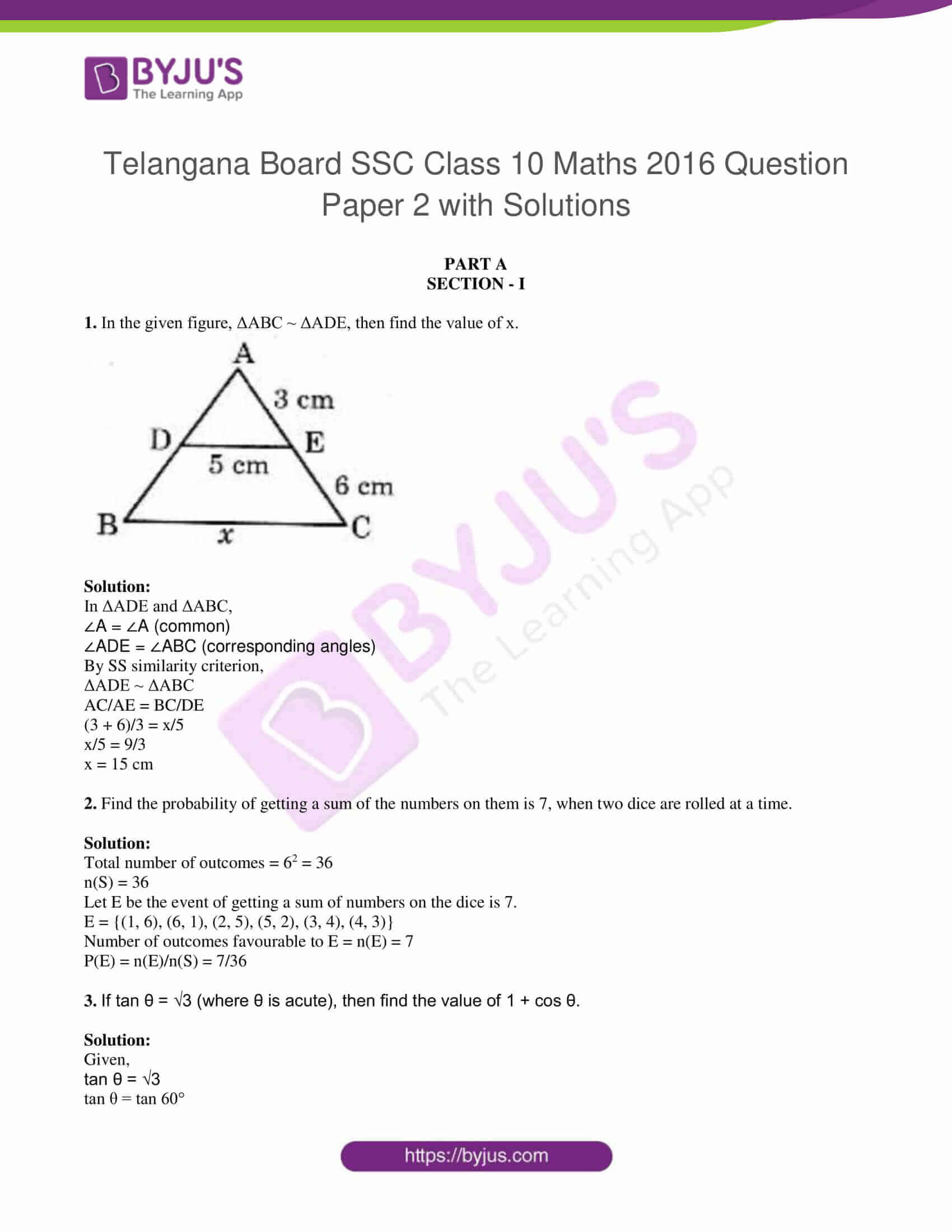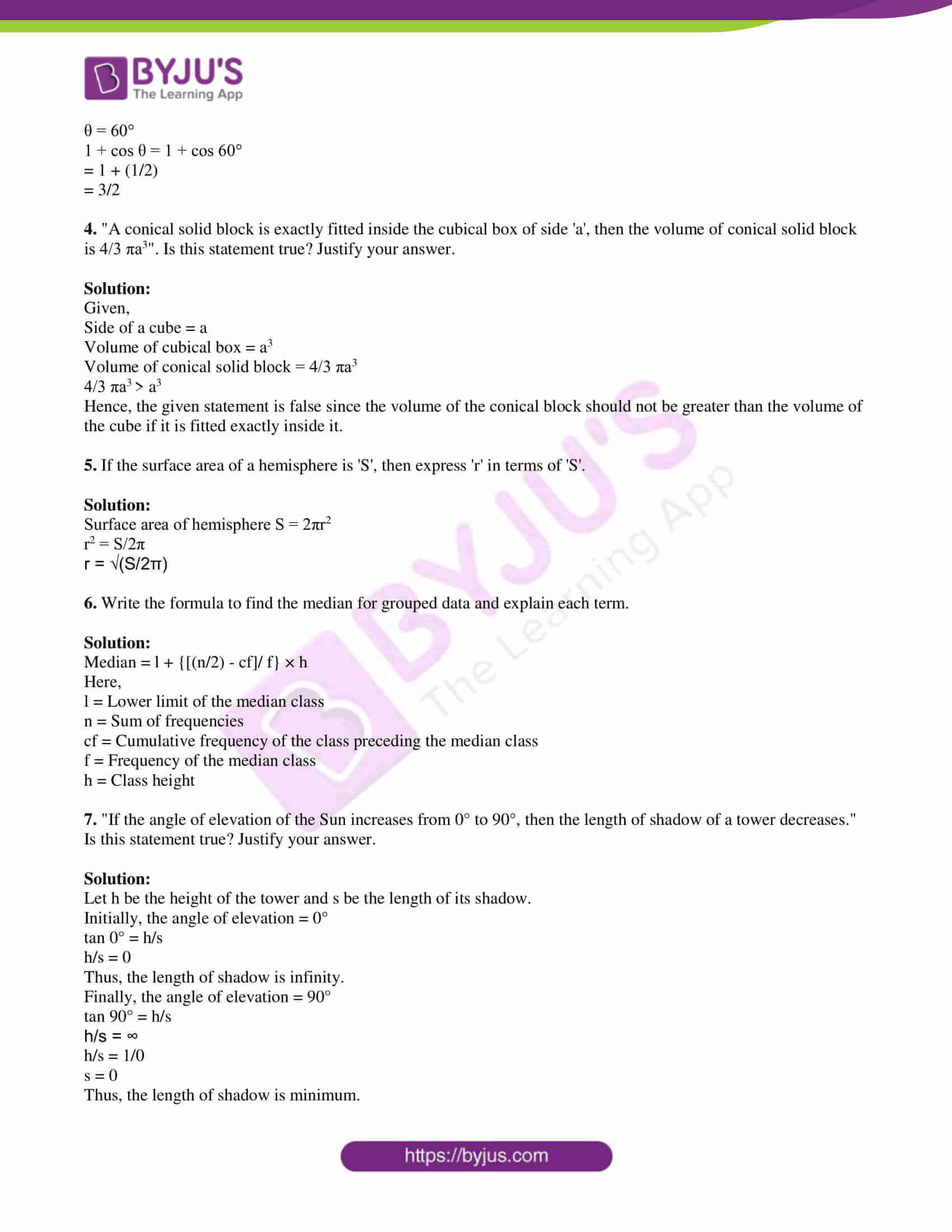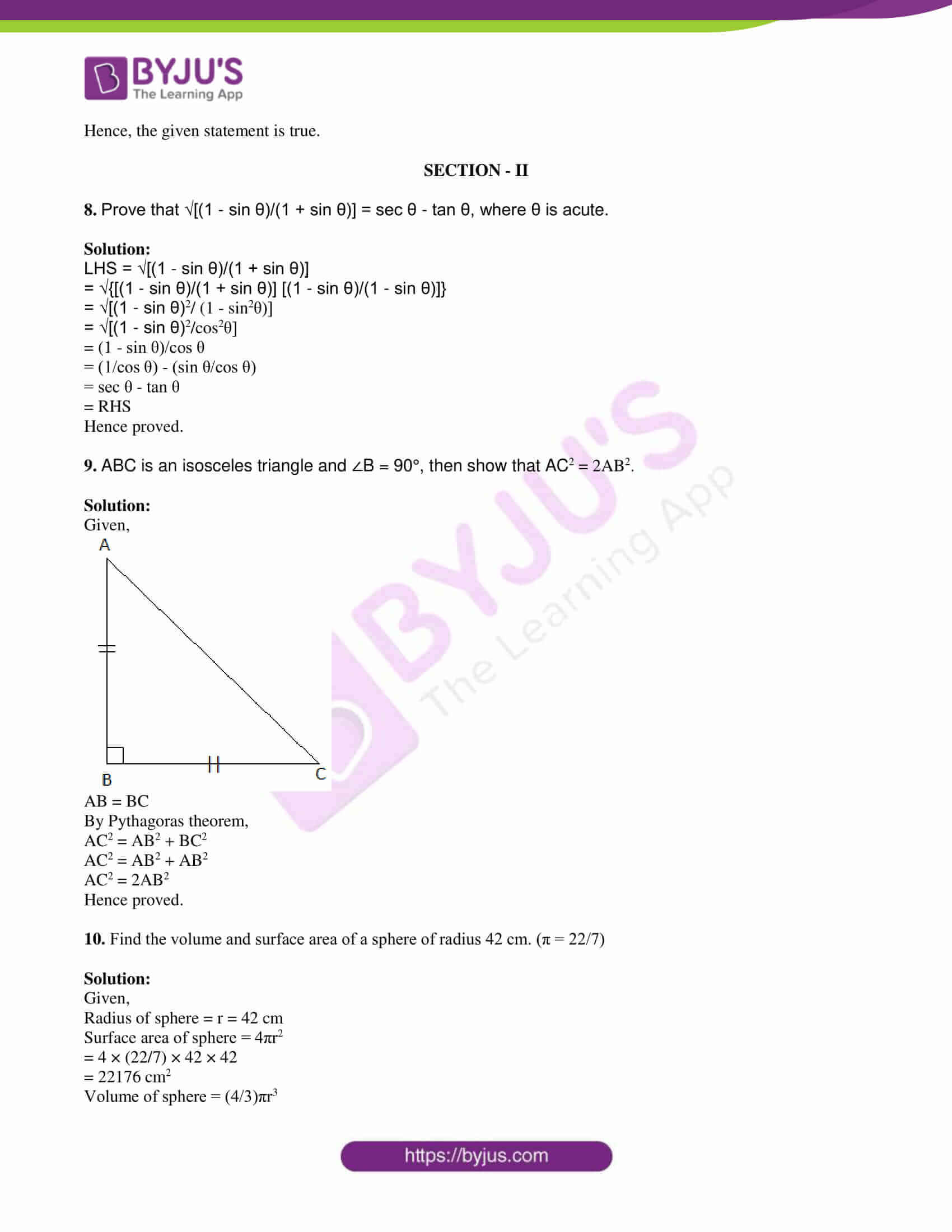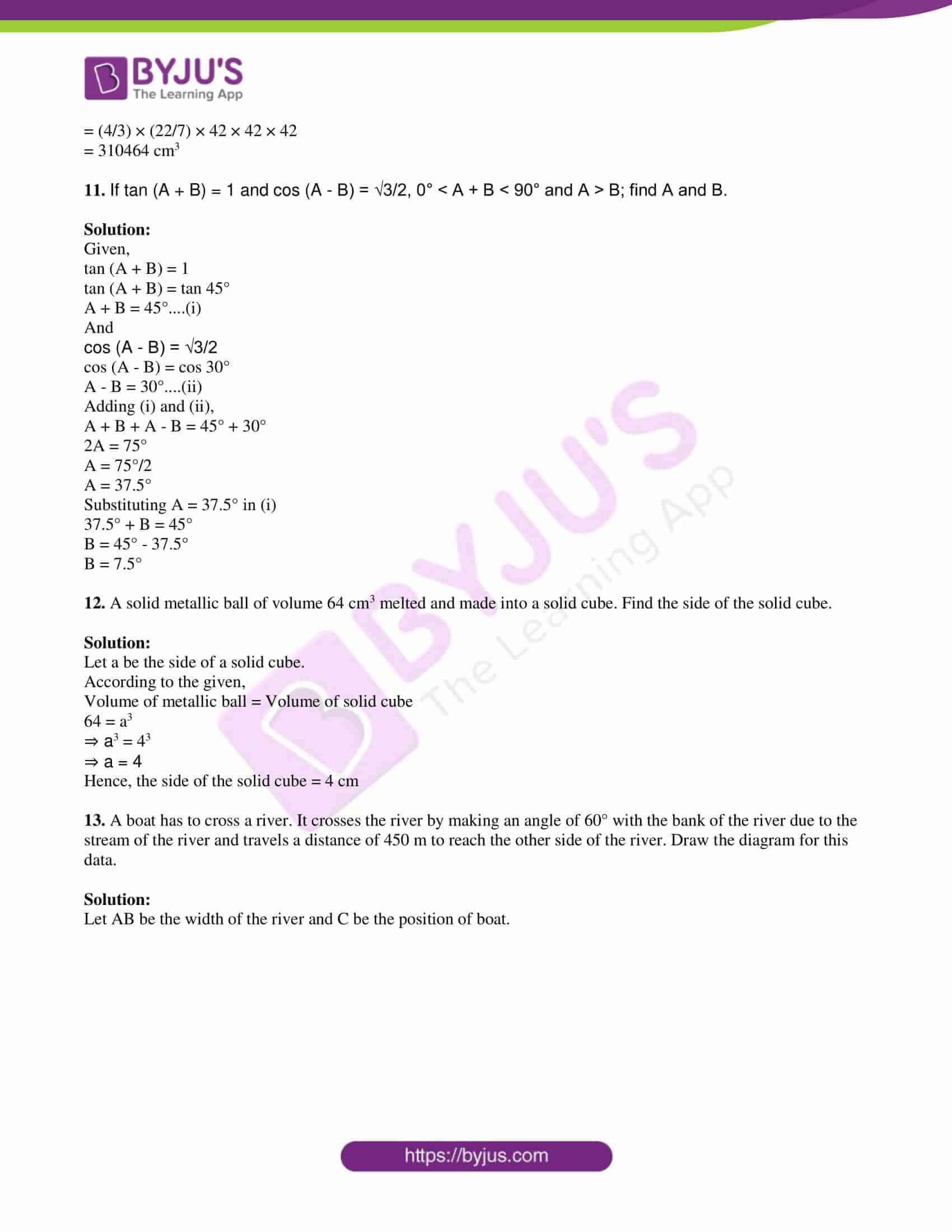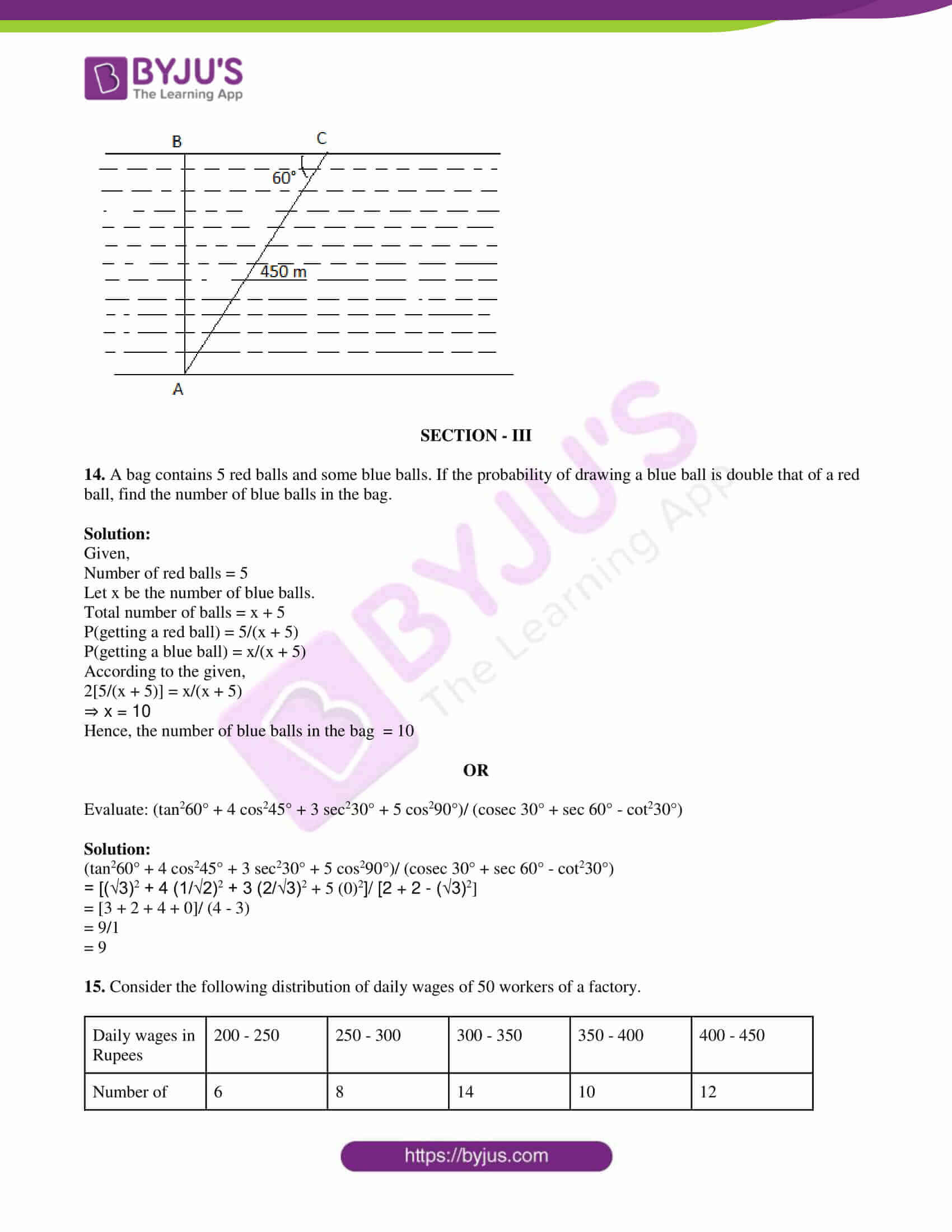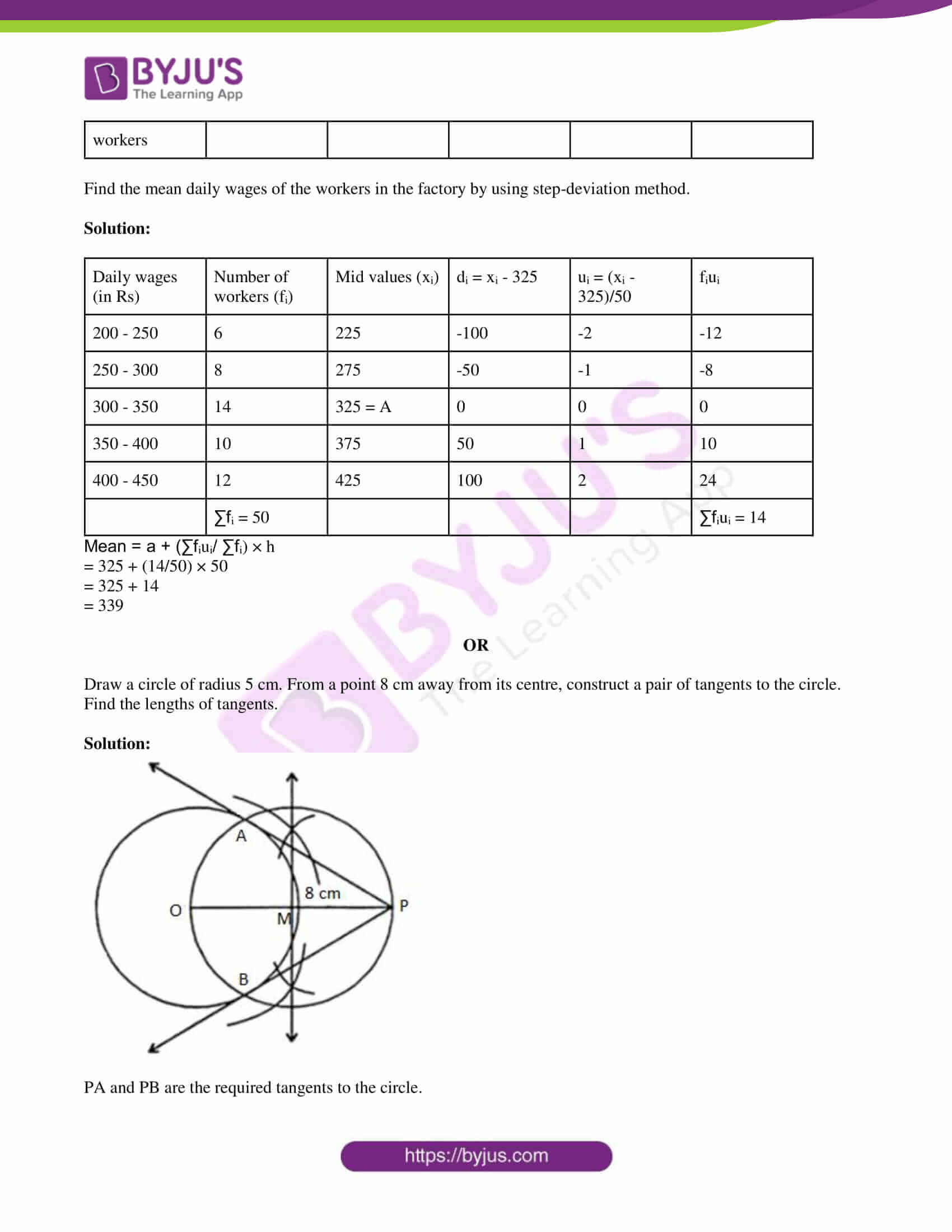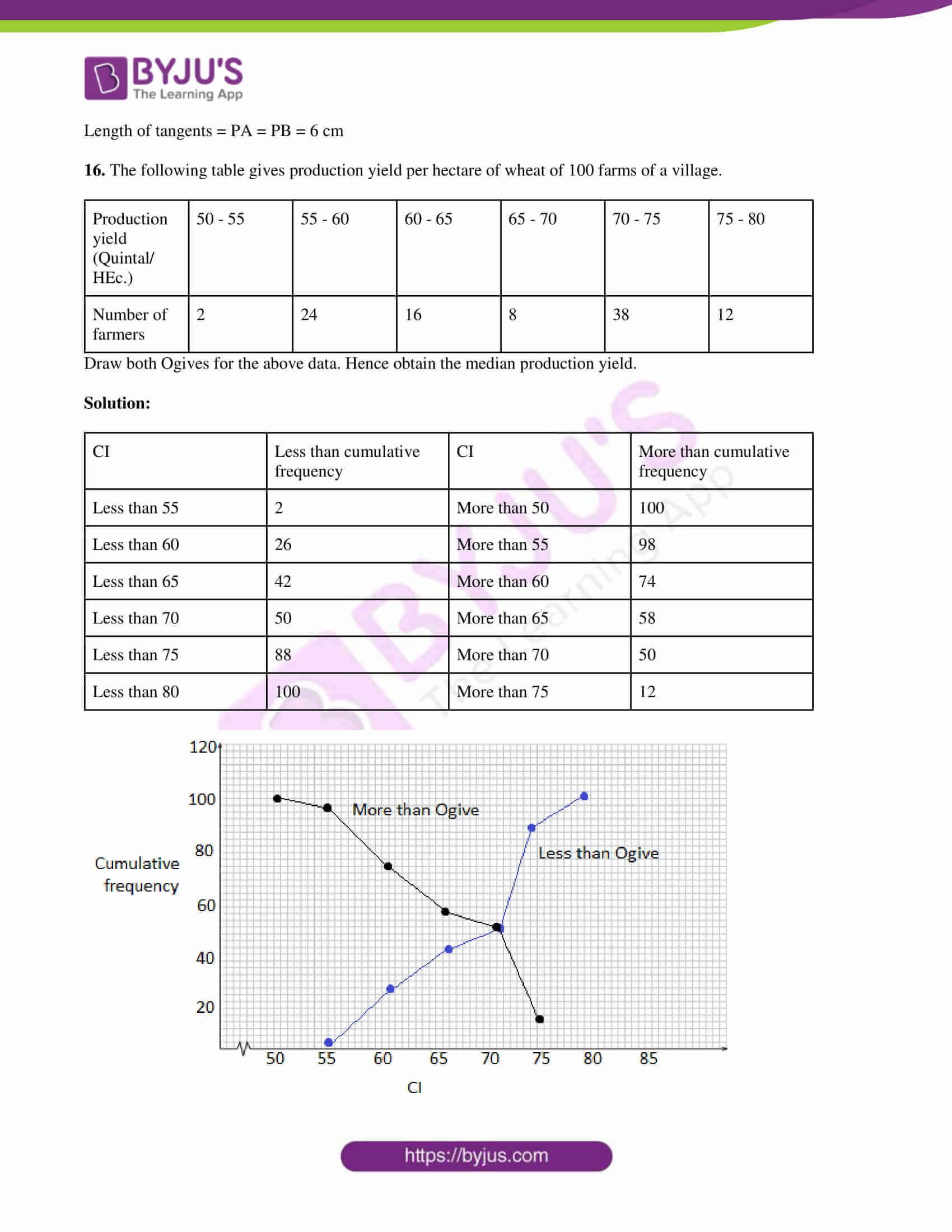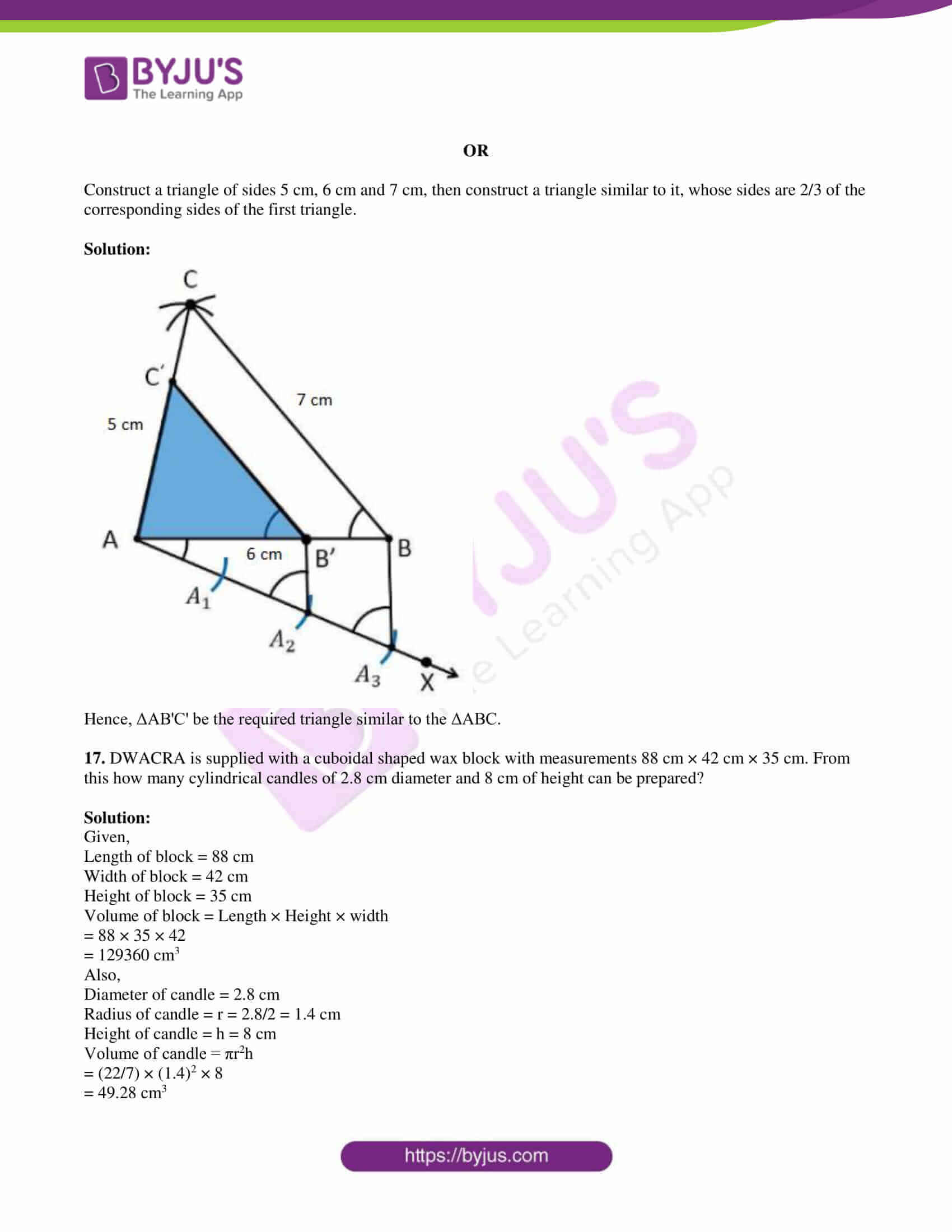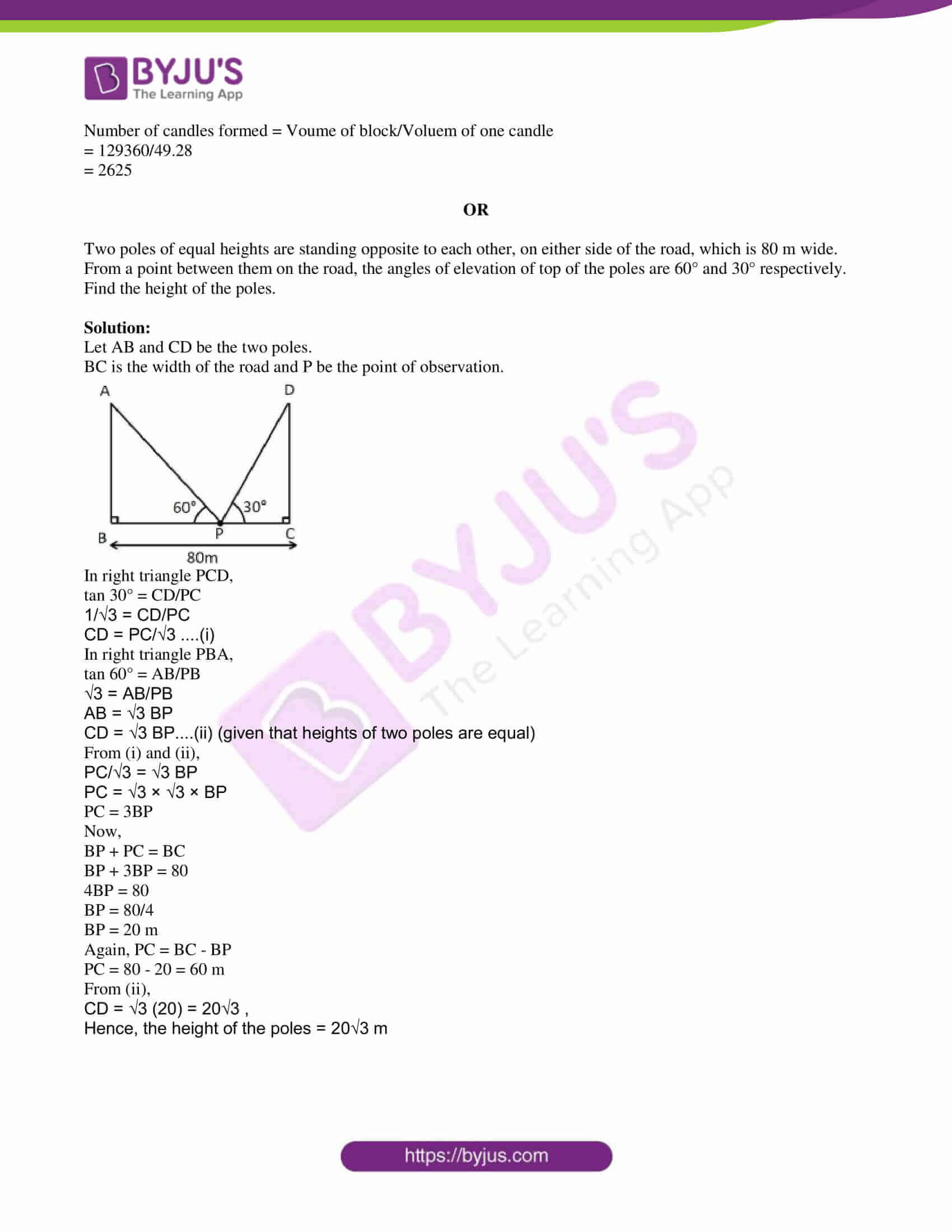PART A

SECTION – I

1. In the given figure, ΔABC ~ ΔADE, then find the value of x.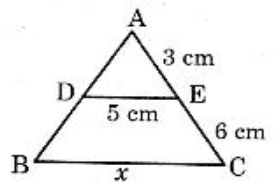Solution:

∠A = ∠A (common)

By SS similarity criterion,

AC/AE = BC/DE

(3 + 6)/3 = x/5

x/5 = 9/3

x = 15 cm

2. Find the probability of getting a sum of the numbers on them is 7, when two dice are rolled at a time.

Solution:

Total number of outcomes = 62 = 36

n(S) = 36

Let E be the event of getting a sum of numbers on the dice is 7.

E = {(1, 6), (6, 1), (2, 5), (5, 2), (3, 4), (4, 3)}

Number of outcomes favourable to E = n(E) = 7

P(E) = n(E)/n(S) = 7/36

3. If tan θ = √3 (where θ is acute), then find the value of 1 + cos θ.

Solution:

Given,

tan θ = √3

tan θ = tan 60°

θ = 60°

1 + cos θ = 1 + cos 60°

= 1 + (1/2)

= 3/2

4. “A conical solid block is exactly fitted inside the cubical box of side ‘a’, then the volume of conical solid block is 4/3 πa3“. Is this statement true? Justify your answer.

Solution:

Given,

Side of a cube = a

Volume of cubical box = a3

Volume of conical solid block = 4/3 πa3

4/3 π3 > a3

Hence, the given statement is false since the volume of the conical block should not be greater than the volume of the cube if it is fitted exactly inside it.

5. If the surface area of a hemisphere is ‘S’, then express ‘r’ in terms of ‘S’.

Solution:

Surface area of hemisphere S = 2πr2

r2 = S/2π

r = √(S/2π)

6. Write the formula to find the median for grouped data and explain each term.

Solution:

Median = l + {[(n/2) – cf]/ f} × h

Here,

l = Lower limit of the median class

n = Sum of frequencies

cf = Cumulative frequency of the class preceding the median class

f = Frequency of the median class

h = Class height

7. “If the angle of elevation of the Sun increases from 0° to 90°, then the length of shadow of a tower decreases.” Is this statement true? Justify your answer.

Solution:

Let h be the height of the tower and s be the length of its shadow.

Initially, the angle of elevation = 0°

tan 0° = h/s

h/s = 0

Thus, the length of shadow is infinity.

Finally, the angle of elevation = 90°

tan 90° = h/s

h/s = ∞

h/s = 1/0

s = 0

Thus, the length of shadow is minimum.

Hence, the given statement is true.

SECTION – II

8. Prove that √[(1 – sin θ)/(1 + sin θ)] = sec θ – tan θ, where θ is acute.

Solution:

LHS = √[(1 – sin θ)/(1 + sin θ)]

= √{[(1 – sin θ)/(1 + sin θ)] [(1 – sin θ)/(1 – sin θ)]}

= √[(1 – sin θ)2/ (1 – sin2θ)]

= √[(1 – sin θ)2/cos2θ]

= (1 – sin θ)/cos θ

= (1/cos θ) – (sin θ/cos θ)

= sec θ – tan θ

= RHS

Hence proved.

9. ABC is an isosceles triangle and ∠B = 90°, then show that AC2 = 2AB2.

Solution:

Given,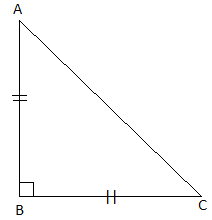AB = BC

By Pythagoras theorem,

AC2 = AB2 + BC2

AC2 = AB2 + AB2

AC2 = 2AB2

Hence proved.

10. Find the volume and surface area of a sphere of radius 42 cm. (π = 22/7)

Solution:

Given,

Radius of sphere = r = 42 cm

Surface area of sphere = 4πr2

= 4 × (22/7) × 42 × 42

= 22176 cm2

Volume of sphere = (4/3)πr3

= (4/3) × (22/7) × 42 × 42 × 42

= 310464 cm3

11. If tan (A + B) = 1 and cos (A – B) = √3/2, 0° < A + B < 90° and A > B; find A and B.

Solution:

Given,

tan (A + B) = 1

tan (A + B) = tan 45°

A + B = 45°….(i)

And

cos (A – B) = √3/2

cos (A – B) = cos 30°

A – B = 30°….(ii)

A + B + A – B = 45° + 30°

2A = 75°

A = 75°/2

A = 37.5°

Substituting A = 37.5° in (i)

37.5° + B = 45°

B = 45° – 37.5°

B = 7.5°

12. A solid metallic ball of volume 64 cm3 melted and made into a solid cube. Find the side of the solid cube.

Solution:

Let a be the side of a solid cube.

According to the given,

Volume of metallic ball = Volume of solid cube

64 = a3

⇒ a3 = 43

⇒ a = 4

Hence, the side of the solid cube = 4 cm

13. A boat has to cross a river. It crosses the river by making an angle of 60° with the bank of the river due to the stream of the river and travels a distance of 450 m to reach the other side of the river. Draw the diagram for this data.

Solution:

Let AB be the width of the river and C be the position of boat.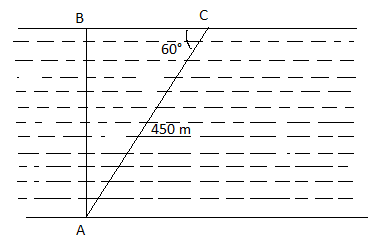SECTION – III

14. A bag contains 5 red balls and some blue balls. If the probability of drawing a blue ball is double that of a red ball, find the number of blue balls in the bag.

Solution:

Given,

Number of red balls = 5

Let x be the number of blue balls.

Total number of balls = x + 5

P(getting a red ball) = 5/(x + 5)

P(getting a blue ball) = x/(x + 5)

According to the given,

2[5/(x + 5)] = x/(x + 5)

⇒ x = 10

Hence, the number of blue balls in the bag = 10

OR

Evaluate: (tan260° + 4 cos245° + 3 sec230° + 5 cos290°)/ (cosec 30° + sec 60° – cot230°)

Solution:

(tan260° + 4 cos245° + 3 sec230° + 5 cos290°)/ (cosec 30° + sec 60° – cot230°)

= [(√3)2 + 4 (1/√2)2 + 3 (2/√3)2 + 5 (0)2]/ [2 + 2 – (√3)2]

= [3 + 2 + 4 + 0]/ (4 – 3)

= 9/1

= 9

15. Consider the following distribution of daily wages of 50 workers of a factory.

 Daily wages in Rupees 200 – 250 250 – 300 300 – 350 350 – 400 400 – 450 Number of workers 6 8 14 10 12

Find the mean daily wages of the workers in the factory by using step-deviation method.

Solution:

 Daily wages (in Rs) Number of workers (fi) Mid values (xi) di = xi – 325 ui = (xi – 325)/50 fiui 200 – 250 6 225 -100 -2 -12 250 – 300 8 275 -50 -1 -8 300 – 350 14 325 = A 0 0 0 350 – 400 10 375 50 1 10 400 – 450 12 425 100 2 24 ∑fi = 50 ∑fiui = 14

Mean = a + (∑fiui/ ∑fi) × h

= 325 + (14/50) × 50

= 325 + 14

= 339

OR

Draw a circle of radius 5 cm. From a point 8 cm away from its centre, construct a pair of tangents to the circle. Find the lengths of tangents.

Solution: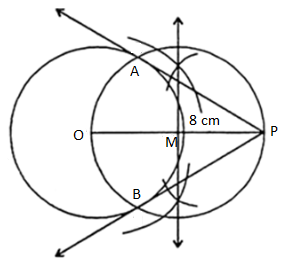PA and PB are the required tangents to the circle.

Length of tangents = PA = PB = 6 cm

16. The following table gives production yield per hectare of wheat of 100 farms of a village.

 Production yield (Quintal/ HEc.) 50 – 55 55 – 60 60 – 65 65 – 70 70 – 75 75 – 80 Number of farmers 2 24 16 8 38 12

Draw both Ogives for the above data. Hence obtain the median production yield.

Solution:

 CI Less than cumulative frequency CI More than cumulative frequency Less than 55 2 More than 50 100 Less than 60 26 More than 55 98 Less than 65 42 More than 60 74 Less than 70 50 More than 65 58 Less than 75 88 More than 70 50 Less than 80 100 More than 75 12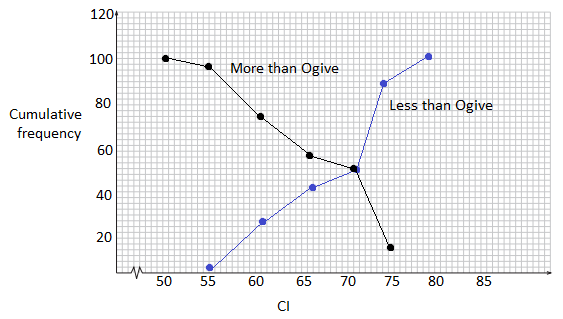OR

Construct a triangle of sides 5 cm, 6 cm and 7 cm, then construct a triangle similar to it, whose sides are 2/3 of the corresponding sides of the first triangle.

Solution: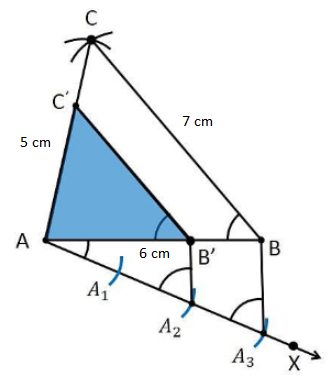Hence, ΔAB’C’ be the required triangle similar to the ΔABC.

17. DWACRA is supplied with a cuboidal shaped wax block with measurements 88 cm × 42 cm × 35 cm. From this how many cylindrical candles of 2.8 cm diameter and 8 cm of height can be prepared?

Solution:

Given,

Length of block = 88 cm

Width of block = 42 cm

Height of block = 35 cm

Volume of block = Length × Height × width

= 88 × 35 × 42

= 129360 cm3

Also,

Diameter of candle = 2.8 cm

Radius of candle = r = 2.8/2 = 1.4 cm

Height of candle = h = 8 cm

Volume of candle = πr2h

= (22/7) × (1.4)2 × 8

= 49.28 cm3

Number of candles formed = Voume of block/Voluem of one candle

= 129360/49.28

= 2625

Hence, the required number of candles is 2625.

OR

Two poles of equal heights are standing opposite to each other, on either side of the road, which is 80 m wide. From a point between them on the road, the angles of elevation of top of the poles are 60° and 30° respectively. Find the height of the poles.

Solution:

Let AB and CD be the two poles.

BC is the width of the road and P be the point of observation.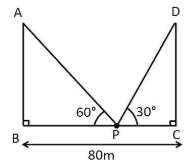In right triangle PCD,

tan 30° = CD/PC

1/√3 = CD/PC

CD = PC/√3 ….(i)

In right triangle PBA,

tan 60° = AB/PB

√3 = AB/PB

AB = √3 BP

CD = √3 BP….(ii) (given that heights of two poles are equal)

From (i) and (ii),

PC/√3 = √3 BP

PC = √3 × √3 × BP

PC = 3BP

Now,

BP + PC = BC

BP + 3BP = 80

4BP = 80

BP = 80/4

BP = 20 m

Again, PC = BC – BP

PC = 80 – 20 = 60 m

From (ii),

CD = √3 (20) = 20√3 ,

Hence, the height of the poles = 20√3 m